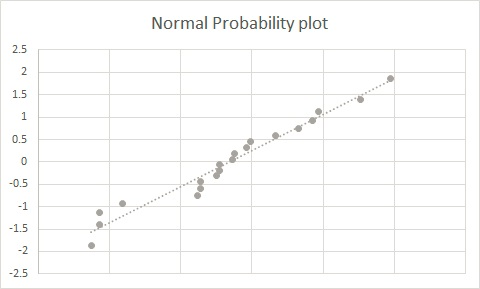The manufacturer of LED lights would like to include as partCheexorgeny 2021-12-19 Answered
The manufacturer of LED lights would like to include as part of their advertising the number of hours an LED is expected to work. A sample of 20 lights revealed the following number of hours worked.
$\begin{array}{|cc|}\hline 2698 & 2628 & 2474 & 2895 & 2672 & 2675 & 2486 & 2852 & 2733 & 2655 \\ 2765 & 2794 & 2486 & 2627 & 2624 & 2785 & 2692 & 2654 & 2518 & 2650 \\ \hline \end{array}$
Part 1: Draw normal probability plot for the above data and make comment.
Part 2: Assuming given data to be approximately normally distributed, Construct 90%, 95% and 99% confidence intervals for the mean number of hours that the LED lights of this brand has worked.
Part 3: Construct 90%, 95% and 99% confidence intervals for the standard deviation of the number of hours that the LED lights of this brand worked.

• Questions are typically answered in as fast as 30 minutes

Solve your problem for the price of one coffee

• Math expert for every subject
• Pay only if we can solve itesfloravaou
Step 1
Introduction:
a) Confidence interval formula for the population mean is given by
$$\displaystyle{C}.{I}=\overline{{{x}}}\pm{z}_{{{\frac{{\alpha}}{{{2}}}}}}{\frac{{{s}{d}}}{{\sqrt{{{n}}}}}}$$
where x-bar is the sample mean
$$\displaystyle{z}_{{{\frac{{\alpha}}{{{2}}}}}}$$ - is the critical value
$$\displaystyle{s}{d}$$ - is the sample standard deviation
$$\displaystyle{n}$$ - is sample size.
b) Confidence interval formula for the population standard deviation is given by
$$\displaystyle{C}.{I}={\frac{{{\left({n}-{1}\right)}{s}^{{{2}}}}}{{{\chi_{{{\frac{{\alpha}}{{{2}}}}}}^{{{2}}}}}}}{ < }\sigma^{{{2}}}{ < }{\frac{{{\left({n}-{1}\right)}{s}^{{{2}}}}}{{{\chi_{{{1}-{\frac{{\alpha}}{{{2}}}}}}^{{{2}}}}}}}$$
Here n is sample size
$$\displaystyle{s}^{{{2}}}$$ - is the sample variance
$$\displaystyle{\chi_{{{\frac{{\alpha}}{{{2}}}}}}^{{{2}}}}$$ - is the critical value
$$\displaystyle{n}$$ - is the sample size
Step 2
Part 1: Normal probability plotFrom the above normal probability plot we can observe that the given set of data follows normal distribution.Since, the all the data points are close to each other and to wards the straight line. Further, it confirms that the number of hours that the LED lights of this brand worked following normality.
Not exactly what you’re looking for?Neil Dismukes
Step 1
Part 2: 90%, 95% and 99% confidence intervals for the mean number of hours that the LED lights of this brand has worked.
For the given data we have,
$$\displaystyle\overline{{{x}}}={2668.15},\ {s}{d}={117.52}$$ and $$\displaystyle{n}={20}$$
Critical value :
$$\displaystyle{z}_{{{\frac{{\alpha}}{{{2}}}}}}={z}_{{{\frac{{{0.1}}}{{{2}}}}}}$$ - is the critical value at $$\displaystyle{n}-{1}={19},\ {d}{f}={1.729}$$
$$\displaystyle{z}_{{{\frac{{\alpha}}{{{2}}}}}}={z}_{{{\frac{{{0.05}}}{{{2}}}}}}$$ - is the critical value at $$\displaystyle{n}-{1}={19},\ {d}{f}={2.093}$$
$$\displaystyle{z}_{{{\frac{{\alpha}}{{{2}}}}}}={z}_{{{\frac{{{0.01}}}{{{2}}}}}}$$ - is the critical value at $$\displaystyle{n}-{1}={19},\ {d}{f}={2.861}$$
90% confidence intervals for the mean number of hours that the LED lights of this brand has worked is
$$\displaystyle{C}.{I}={2668.15}\pm{1.729}\times{\frac{{{117.52}}}{{\sqrt{{{20}}}}}}$$
$$\displaystyle={2668.15}\pm{45.44}$$
$$\displaystyle\text{Lower limit}={2668.15}-{45.44}={2622.71}$$
$$\displaystyle\text{Upper limit}={2668.15}+{45.44}={2713.59}$$
So,we are 90% confident that the mean number of hours that the LED lights of this brand has worked is lies between 2622.71 and 2713.59
95% confidence intervals for the mean number of hours that the LED lights of this brand has worked is
$$\displaystyle{C}.{I}={2668.15}\pm{2.093}\times{\frac{{{117.52}}}{{\sqrt{{{20}}}}}}$$
$$\displaystyle={2668.15}\pm{55}$$
$$\displaystyle\text{Lower limit}={2668.15}-{55}={2613.15}$$
$$\displaystyle\text{Upper limit}={2668.15}+{55}={2723.15}$$
So,we are 95% confident that the mean number of hours that the LED lights of this brand has worked is lies between 2613.15 and 2713.15.
99% confidence intervals for the mean number of hours that the LED lights of this brand has worked is
$$\displaystyle{C}.{I}={2668.15}\pm{2.861}\times{\frac{{{117.52}}}{{\sqrt{{{20}}}}}}$$
$$\displaystyle={2668.15}\pm{75.18}$$
$$\displaystyle\text{Lower limit}={2668.15}-{75.18}={2592.97}$$
$$\displaystyle\text{Upper limit}={2668.15}+{75.18}={2743.33}$$
So,we are 99% confident that the mean number of hours that the LED lights of this brand has worked is lies between 2592.97 and 2743.33.nick1337

Step 1
Part 3: 90%, 95% and 99% confidence intervals for the standard deviation of the number of hours that the LED lights of this brand worked.
For the given data we have,
$$\bar{x}=266.15$$

sd=117.52
n=20
Critical value :
$$\chi_{1-\frac{\alpha}{2}}^{2}=\chi_{0.90,\ 19}^{2}=11.6509$$ and
$$\chi_{\frac{\alpha}{2}}^{2}=\chi_{0.1,\ 19}^{2}=27.2036$$
$$\chi_{1-\frac{\alpha}{2}}^{2}=\chi_{0.95,\ 19}^{2}=10.1170$$ and
$$\chi_{\frac{\alpha}{2}}^{2}=\chi_{0.05,\ 19}=30.1435$$
$$\chi_{1-\frac{\alpha}{2}}^{2}=\chi_{0.99,\ 19}^{2}=7.6327$$ and
$$\chi_{\frac{\alpha}{2}}^{2}=\chi_{0.01,\ 19}^{2}=36.1909$$
90% confidence intervals for the standard deviation of the number of hours that the LED lights of this brand worked.
$$C.I=\frac{(20-1)117.52^{2}}{27.2036}<\sigma^{2}<\frac{(20-1)117.52^{2}}{11.6509}$$
$$=9646.327<\sigma^{2}<22523.09$$
$$=98.21<\sigma<150.077$$
So,we are 90% confident that the Standard deviation of hours that the LED lights of this brand has worked is lies between 98.21 and 150.077
95% confidence intervals for the standard deviation of the number of hours that the LED lights of this brand worked.
$$C.I=\frac{(20-1)117.52^{2}}{30.1435}<\sigma^{2}<\frac{(20-1)117.52^{2}}{10.1170}$$
$$=8705.502<\sigma^{2}<25937.95$$
$$=93.30<\sigma<161.05$$
So,we are 95% confident that the Standard deviation of hours that the LED lights of this brand has worked is lies between 93.30 and 161.05
99% confidence intervals for the standard deviation of the number of hours that the LED lights of this brand worked.
$$C.I=\frac{(20-1)117.52^{2}}{36.1909}<\sigma^{2}<\frac{(20-1)117.52^{2}}{7.6327}$$
$$=7250.85<\sigma^{2}<34380.17$$
$$=85.15<\sigma<185.41$$
So,we are 99% confident that the Standard deviation of hours that the LED lights of this brand has worked is lies between 85.15and 185.41